# NumPy Array manipulation: flip() function

## numpy.flip() function

The flip() function is used to reverse the order of elements in an array along the given axis.
The shape of the array is preserved, but the elements are reordered.

Syntax:

`numpy.flip(m, axis=None)`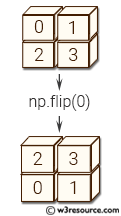Version: 1.15.0

Parameter:

Name Description Required /
Optional
m Input array. Required
axis Axis or axes along which to flip over. The default, axis=None, will flip over all of the axes of the input array. If axis is negative it counts from the last to the first axis.
If axis is a tuple of ints, flipping is performed on all of the axes specified in the tuple.
Optional

Return value:

out : array_like - A view of m with the entries of axis reversed. Since a view is returned, this operation is done in constant time.

Example-1: numpy.flip()

``````>>> import numpy as np
>>> X = np.arange(8).reshape((2,2,2))
>>> X
array([[[0, 1],
[2, 3]],

[[4, 5],
[6, 7]]])
``````

Pictorial Presentation: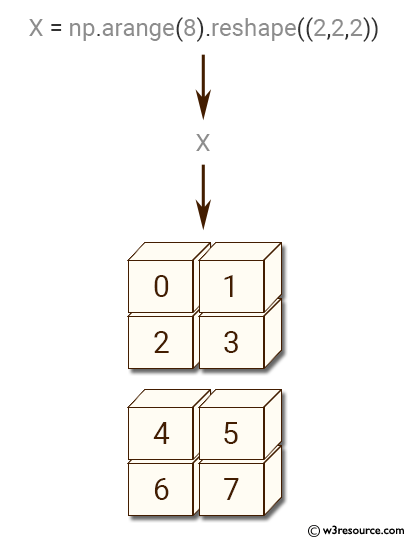Example-2: numpy.flip()

``````>>> import numpy as np
>>> X = np.arange(8).reshape((2,2,2))
>>> np.flip(X, 0)
array([[[4, 5],
[6, 7]],
[[0, 1],
[2, 3]]])
>>> np.flip(X, 1)
array([[[2, 3],
[0, 1]],
[[6, 7],
[4, 5]]])
>>> np.flip(X)
array([[[7, 6],
[5, 4]],
[[3, 2],
[1, 0]]])
>>> np.flip(X, (0, 2))
array([[[5, 4],
[7, 6]],
[[1, 0],
[3, 2]]])
>>> X = np.random.randn(3,4,5)
>>> np.all(flip(X,2) == X[:,:,::-1,...])
True
``````

Pictorial Presentation: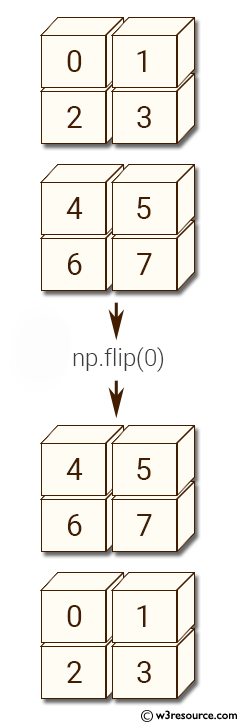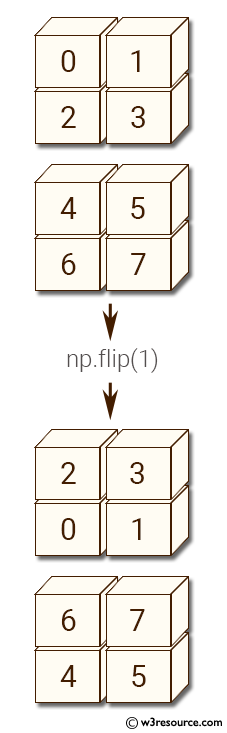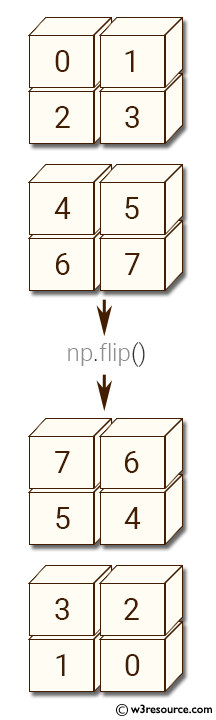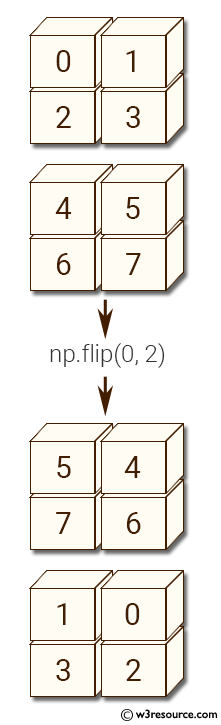Python - NumPy Code Editor:

Previous: unique()
Next: fliplr()

﻿